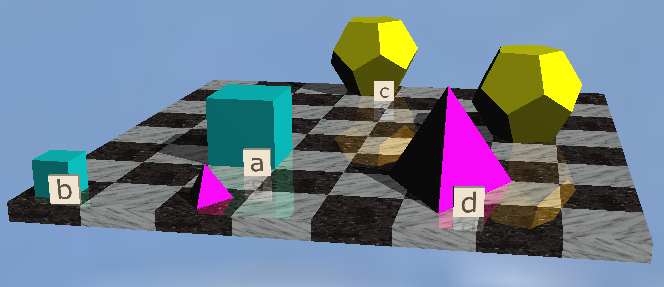# LP&L Trees 5 Predicate Samples

Logical System
###### 1/28/2020

John Barwise and John Etchemendy,  Language, Proof and Logic

For the Tarski's World part of LP&L, a standard interpretation is used of various sized polyhedra on a chessboard. Here is a link to their screenshot of it Tarski's World.Here we are just trying a few samples to see if the software is running correctly.

## Exercise

[In the widget below:- Click once to select, click again to un-select. Select one item to extend the Tree, select two to close a branch. Select one or two for extending by Analytic Consequence (depending what inference you are trying for). Select two for extending with identity.]

1. ∀xCube(x)→∃ySmall(y), ¬∃ySmall(y), ∴ ¬∀xCube(x) (p.265)

2. ∴ ∃w(Dodec(w)∧Large(w)) ↔ (∃wDodec(w)∧∃wLarge(w)) (p.283) Not valid the tree will stay open.

3. ∀x∀y(LeftOf(x,y)→Larger(x,y)), ∀x(Cube(x)→Small(x)), ∀x(Tet(x)→Large(x)), ∀x∀y((Small(x)∧Small(y))→¬Larger(x,y)) ∴ ¬∃x∃y(Cube(x)∧Cube(y)∧ RightOf(x,y)) (p.336) May or may not be valid [Hint: it is valid but you don't need the Tet premise.]